• 【图像算法】引导滤波matlab代码
千次阅读
2020-11-16 16:25:26

写作时间：2020-11-16
目录：

正文：
引导滤波，又称为导向滤波。下面为代码，包含一个主函数guidedfilter和一个子函数boxfilter。
（1）主函数

function q = guidedfilter(I, p, r, eps)
%   GUIDEDFILTER   O(1) time implementation of guided filter.
%
%   - guidance image导向滤波
更多相关内容
• 导向滤波matlab代码相关合集，包含增强算法，羽化算法，滤波平滑算法等。导向滤波（Guided Filtering）和双边滤波（BF）、最小二乘滤波（WLS）是三大边缘保持（Edge-perserving）滤波器。当然，引导滤波的功能不仅仅...
• 混合滤波matlab代码 StuctureOrientedFilter_3D 使用结构导向滤波处理三维地震数据(也可以处理三通道彩色图片) 输入/输出 matlab .mat格式 代码环境 armadillo-9.600.6 / matlab C++混合编程 / 未使用openCV 数据...
• 何恺明导向滤波器MATLAB源码--guidedfilter.m matlab图像处理小白文件锦集，用最短的时间找到最有用的资源。 文件真实有效。
• http://blog.csdn.net/kuweicai/article/details/783858711. 导向滤波简介导向滤波是何凯明在学生时代提出的一个保边滤波(edge-preserving smoothing)算法。何凯明在cv圈应该算是名人了，学生时代关于图像去雾的研究...

http://blog.csdn.net/kuweicai/article/details/78385871

1. 导向滤波简介

导向滤波是何凯明在学生时代提出的一个保边滤波(edge-preserving smoothing)算法。何凯明在cv圈应该算是名人了，学生时代关于图像去雾的研究就以第一作者的身份获得Best Paper Award(CVPR 2009)，而且今年刚刚又斩获Marr Prize(ICCV 2017)。更多关于何凯明的最新研究动态可以点击以下链接何凯明。

导向滤波顾名思义，就是有选择(导向)性的滤波，其与我们经常提及的高斯滤波、双边滤波相比，它具有导向性，说具体点就是，它通过输入一副图像(矩阵)作为导向图，这样滤波器就知道什么地方是边缘，这样就可以更好的保护边缘，最终达到在滤波的同时，保持边缘细节。所以有个说法是导向滤波是各向异性的滤波器，而高斯滤波、双边滤波这些是各向同性滤波器，我觉得也是很贴切。

导向滤波作为一种保边滤波，可以运用在很多场合，比如美颜，去雾，三维重建等。

如果你仅仅只是需要运用这个算法，现在opencv 3.0和MATLAB 14都已经添加了guided filter的API，可以直接调用。

opencv中的API如下void cv::ximgproc::guidedFilter()，具体的可以参考opencv的帮助文档关于导向滤波的介绍guidedFilter。

但是需要提醒的是，opencv中guidedFilter()函数包含在ximgproc模块下，但是从官方下载的标准的opencv.exe程序中并不包含该模块，需要分别下载opencv的source文件和contrib模块的source文件，然后自己编译，具体可以参考opencv3.1.0+contrib模块编译总结。

2. 导向滤波的原理

查看了很多相关的资料，觉得白马负金羁的导向滤波(Guided Filter)的解析与实现一文将其原理解释的非常通俗易懂了，这里就不再赘述。仅给出最后的推导结果，其中fmean为一个窗口半径为r的均值滤波器(对应的窗口大小为2r+1)，corr为相关，var为方差，cov为协方差。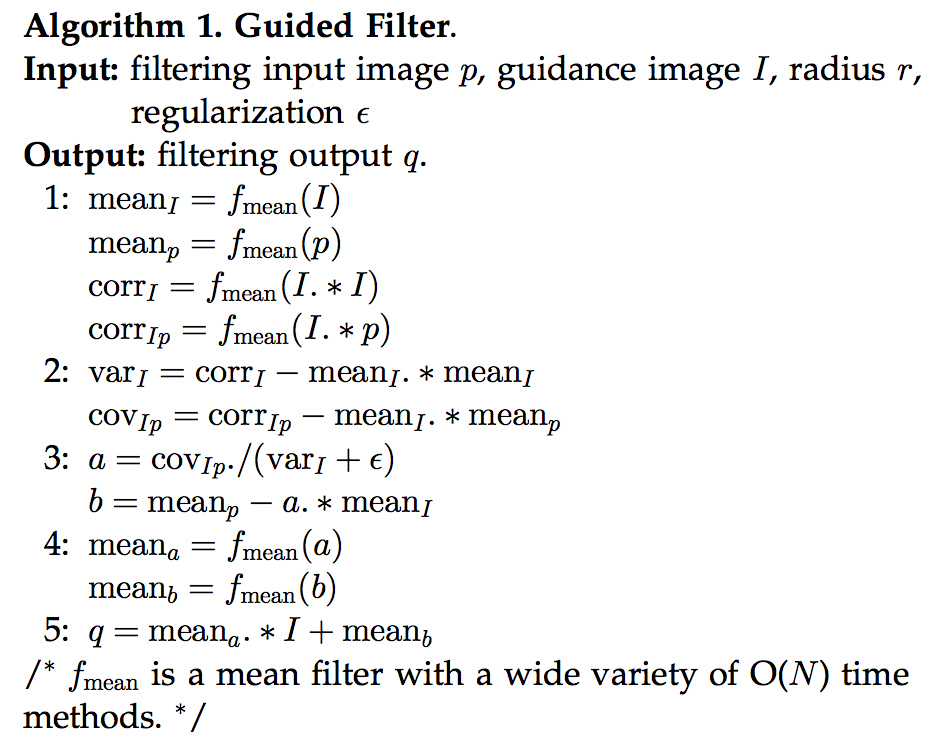3. opencv实现代码

这一部分主要参考了

OpenCV导向滤波(引导滤波)实现(Guided Filter)代码，以及使用颜色先验算法去雾中的代码，进行了修改和注释。GuidedFilter()调用opencv自带的boxFilter()函数来实现求取平均值。关于opencv自带的boxFilter()函数的相关介绍可以参考

boxFilter。

GuidedFilter()的代码，比较容易理解：

cv::Mat GuidedFilter(cv::Mat I,cv::Mat p,intr,doubleeps)

{

/*

% GUIDEDFILTER   O(N) time implementation of guided filter.

%

%   - guidance image: I (should be a gray-scale/single channel image)

%   - filtering input image: p (should be a gray-scale/single channel image)

%   - local window radius: r

%   - regularization parameter: eps

*/

cv::Mat _I;

I.convertTo(_I, CV_64FC1,1.0/255);

I = _I;

cv::Mat _p;

p.convertTo(_p, CV_64FC1,1.0/255);

p = _p;

//[hei, wid] = size(I);

inthei = I.rows;

intwid = I.cols;

r=2*r+1;//因为opencv自带的boxFilter()中的Size,比如9x9,我们说半径为4

//mean_I = boxfilter(I, r) ./ N;

cv::Mat mean_I;

cv::boxFilter(I, mean_I, CV_64FC1,cv::Size(r, r));

//mean_p = boxfilter(p, r) ./ N;

cv::Mat mean_p;

cv::boxFilter(p, mean_p, CV_64FC1,cv::Size(r, r));

//mean_Ip = boxfilter(I.*p, r) ./ N;

cv::Mat mean_Ip;

cv::boxFilter(I.mul(p), mean_Ip, CV_64FC1,cv::Size(r, r));

//cov_Ip = mean_Ip - mean_I .* mean_p; % this is the covariance of (I, p) in each local patch.

cv::Mat cov_Ip = mean_Ip - mean_I.mul(mean_p);

//mean_II = boxfilter(I.*I, r) ./ N;

cv::Mat mean_II;

cv::boxFilter(I.mul(I), mean_II, CV_64FC1,cv::Size(r, r));

//var_I = mean_II - mean_I .* mean_I;

cv::Mat var_I = mean_II - mean_I.mul(mean_I);

//a = cov_Ip ./ (var_I + eps); % Eqn. (5) in the paper;

cv::Mat a = cov_Ip / (var_I + eps);

//b = mean_p - a .* mean_I; % Eqn. (6) in the paper;

cv::Mat b = mean_p - a.mul(mean_I);

//mean_a = boxfilter(a, r) ./ N;

cv::Mat mean_a;

cv::boxFilter(a, mean_a, CV_64FC1,cv::Size(r, r));

//mean_b = boxfilter(b, r) ./ N;

cv::Mat mean_b;

cv::boxFilter(b, mean_b, CV_64FC1,cv::Size(r, r));

//q = mean_a .* I + mean_b; % Eqn. (8) in the paper;

cv::Mat q = mean_a.mul(I) + mean_b;

returnq;

}

需要注意的是，上面的函数只能对单一通道进行处理(如果是多通道，需要split后进行滤波，然后merge)。下面是调用GuidedFilter()，r=16, eps=0.01，对原图像进行滤波的结果。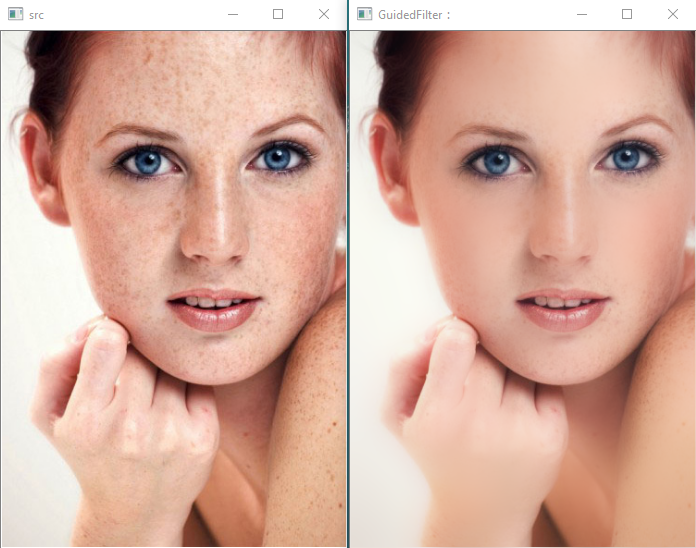4. 快速导向滤波

导向滤波的时间复杂度为O(N)，其中N为像素点的个数。

何凯明在2015又发表了一篇《Fast Guided Filter》的文章，阐述了一种很实用的更快速的导向滤波流程。如下所示。其本质是通过下采样减少像素点，计算mean_a & mean_b后进行上采样回复到原有的尺寸大小。假设缩放比例为s,那么缩小后像素点的个数为N/s^2，那么时间复杂度变为O(N/s^2)(只是需要注意的是上采样和下采样本身也是有时间消化的)。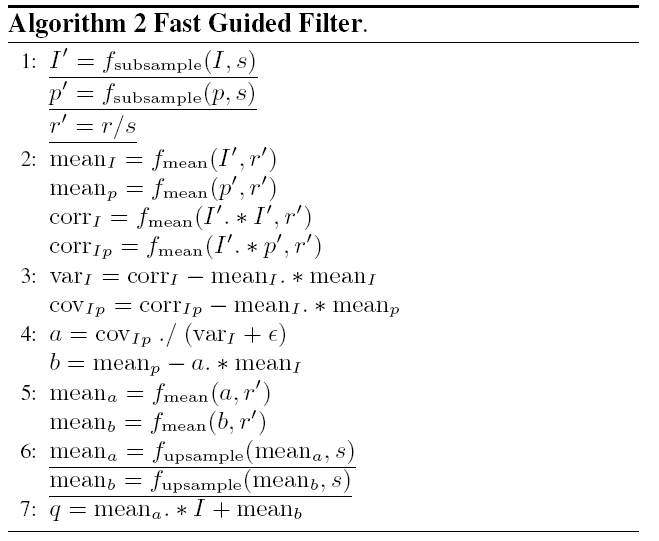基于上面的理论，只需调用resize()函数来实现下采样和上采样，关于resize()函数的使用可以参考

resize()代码如下：

cv::Mat fastGuidedFilter(cv::Mat I_org,cv::Mat p_org,intr,doubleeps,ints)

{

/*

% GUIDEDFILTER   O(N) time implementation of guided filter.

%

%   - guidance image: I (should be a gray-scale/single channel image)

%   - filtering input image: p (should be a gray-scale/single channel image)

%   - local window radius: r

%   - regularization parameter: eps

*/

cv::Mat I,_I;

I_org.convertTo(_I, CV_64FC1,1.0/255);

resize(_I,I,Size(),1.0/s,1.0/s,1);

cv::Mat p,_p;

p_org.convertTo(_p, CV_64FC1,1.0/255);

//p = _p;

resize(_p, p, Size(),1.0/s,1.0/s,1);

//[hei, wid] = size(I);

inthei = I.rows;

intwid = I.cols;

r = (22* r +1)/s+1;//因为opencv自带的boxFilter()中的Size,比如9x9,我们说半径为4

//mean_I = boxfilter(I, r) ./ N;

cv::Mat mean_I;

cv::boxFilter(I, mean_I, CV_64FC1,cv::Size(r, r));

//mean_p = boxfilter(p, r) ./ N;

cv::Mat mean_p;

cv::boxFilter(p, mean_p, CV_64FC1,cv::Size(r, r));

//mean_Ip = boxfilter(I.*p, r) ./ N;

cv::Mat mean_Ip;

cv::boxFilter(I.mul(p), mean_Ip, CV_64FC1,cv::Size(r, r));

//cov_Ip = mean_Ip - mean_I .* mean_p; % this is the covariance of (I, p) in each local patch.

cv::Mat cov_Ip = mean_Ip - mean_I.mul(mean_p);

//mean_II = boxfilter(I.*I, r) ./ N;

cv::Mat mean_II;

cv::boxFilter(I.mul(I), mean_II, CV_64FC1,cv::Size(r, r));

//var_I = mean_II - mean_I .* mean_I;

cv::Mat var_I = mean_II - mean_I.mul(mean_I);

//a = cov_Ip ./ (var_I + eps); % Eqn. (5) in the paper;

cv::Mat a = cov_Ip / (var_I + eps);

//b = mean_p - a .* mean_I; % Eqn. (6) in the paper;

cv::Mat b = mean_p - a.mul(mean_I);

//mean_a = boxfilter(a, r) ./ N;

cv::Mat mean_a;

cv::boxFilter(a, mean_a, CV_64FC1,cv::Size(r, r));

Mat rmean_a;

resize(mean_a, rmean_a, Size(I_org.cols, I_org.rows),1);

//mean_b = boxfilter(b, r) ./ N;

cv::Mat mean_b;

cv::boxFilter(b, mean_b, CV_64FC1,cv::Size(r, r));

Mat rmean_b;

resize(mean_b, rmean_b, Size(I_org.cols, I_org.rows),1);

//q = mean_a .* I + mean_b; % Eqn. (8) in the paper;

cv::Mat q = rmean_a.mul(_I) + rmean_b;

returnq;

}

取s==8，计算结果和之前的guidedFilter()的计算结果如下所示：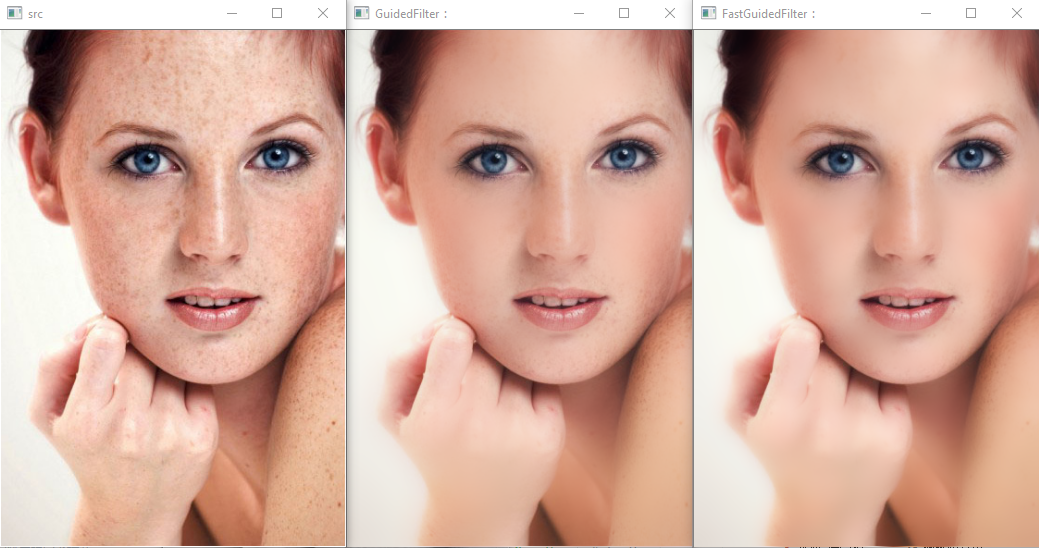而计算时间却从 338.808ms降到100.856ms，但是滤波结果从肉眼观察几乎没有降低。

展开全文• 导向滤波算法实现图像去雾，可以直接运行。。。。。。
• 高斯低通滤波matlab代码OptDSP库的一般说明 OptDSP库包含一组m文件，以实现用于相干光通信系统的发射机和接收机侧数字信号处理（DSP） 。 当前支持的功能： 脉冲整形（RRC，SRRC，高斯等）; QAM调制（BPSK，QPSK，8...
• 无论是简单平滑，还是高斯平滑，它们都有一个共同的弱点，即它们都属于各向同性滤波。我们都知道，一幅自然的图像可以被看成是有（过渡平缓的，也就是梯度较小）区域和（过渡尖锐的，也就是梯度较大）边缘（也包括...

现在从一个最简单的情形来开始我们的讨论。假设有一个原始图像 pp较远的像素则具有更小的权重。

无论是简单平滑，还是高斯平滑，它们都有一个共同的弱点，即它们都属于各向同性滤波。我们都知道，一幅自然的图像可以被看成是有（过渡平缓的，也就是梯度较小）区域和（过渡尖锐的，也就是梯度较大）边缘（也包括图像的纹理、细节等）共同组成的。噪声是影响图像质量的不利因素，我们希望将其滤除。噪声的特点通常是以其为中心的各个方向上梯度都较大而且相差不多。边缘则不同，边缘相比于区域也会出现梯度的越变，但是边缘只有在其法向方向上才会出现较大的梯度，而在切向方向上梯度较小。

因此，对于各向同性滤波（例如简单平滑或高斯平滑）而言，它们对待噪声和边缘信息都采取一直的态度。结果，噪声被磨平的同时，图像中具有重要地位的边缘、纹理和细节也同时被抹平了。这是我们所不希望看到的。研究人员已经提出了很多Edge-perserving的图像降噪（平滑）算法，例如双边滤波、自适应（维纳）平滑滤波（请参见文献【1】）、基于PM方程的各向异性滤波以及基于TV-norm的降噪算法等。本文将考虑在文献【2】中提出的另外一种Edge-perserving的图像滤波（平滑）算法——导向滤波（Guided Filter）。当然，通过阅读文献【2】，我们也知道导向滤波的应用不止有Edge-perserving的图像平滑，还包括图像去雾、图像Matting等等。

欢迎关注白马负金羁的博客 http://blog.csdn.net/baimafujinji，为保证公式、图表得以正确显示，强烈建议你从该地址上查看原版博文。本博客主要关注方向包括：数字图像处理、算法设计与分析、数据结构、机器学习、数据挖掘、统计分析方法、自然语言处理。

## 算法原理

导向滤波之所以叫这个名字，因为在算法框架中，要对pp本身的时候，导向滤波就变成了一个Edge-perserving的滤波器，因此可以用于图像的平滑降噪。

导向滤波滤波的示意图如下所示（图片来自作者原文【2】）。注意这也是导向滤波所依赖的一个重要假设——导向滤波器在导向图像II中，尽管丙处的梯度仍然大于乙处的梯度，进而大于甲处的梯度，但是倍数关系可能会扭曲。例如，丙处的梯度是乙处的1.5倍，而是甲处的6倍（即乙处的梯度是甲处的4倍），这时你就会想象，甲处是区域，而乙处和丙处就变成了边缘。可见非线性关系会使得引导图像对于边缘和区域的指示作用发生错乱。

现在已知的是II），corr为相关，var为方差，cov为协方差。## 基于MATLAB的算法实现

下面来在MATLAB中具体实现一下导向滤波算法（代码来自Dr. Kaiming He，使用时请尊重原作者权利）。

function q = guidedfilter(I, p, r, eps)

%   - guidance image: I (should be a gray-scale/single channel image)
%   - filtering input image: p (should be a gray-scale/single channel image)
%   - local window radius: r
%   - regularization parameter: eps

[hei, wid] = size(I);
N = boxfilter(ones(hei, wid), r);

mean_I = boxfilter(I, r) ./ N;
mean_p = boxfilter(p, r) ./ N;
mean_Ip = boxfilter(I.*p, r) ./ N;
% this is the covariance of (I, p) in each local patch.
cov_Ip = mean_Ip - mean_I .* mean_p;

mean_II = boxfilter(I.*I, r) ./ N;
var_I = mean_II - mean_I .* mean_I;

a = cov_Ip ./ (var_I + eps);
b = mean_p - a .* mean_I;

mean_a = boxfilter(a, r) ./ N;
mean_b = boxfilter(b, r) ./ N;

q = mean_a .* I + mean_b;
end
123456789101112131415161718192021222324252627

上述代码的实现完全遵照上一小节最后给出的算法流程图。这里需要略作解释的地方是函数boxfilter，它是基于积分图算法实现的Box Filter。关于Box Filter，你也可以参考文献【6】以了解更多。首先来看看它到底做了些什么（因为这个Box Filter 和通常意义上的均值滤波并不完全一样）。

A =

1     1     1
1     1     1
1     1     1

>> B = boxfilter(A, 1);
>> B

B =

4     6     4
6     9     6
4     6     4
1234567891011121314

从上述代码可以看到，当参数r=1时，此时的滤波器窗口是3×33×3。将这样大小的一个窗口扣在原矩阵中心，刚好可以覆盖所有矩阵，此时求和为9，即把窗口里覆盖到的值全部加和。此外当把窗口中心挪动到左上角的像素时，因为窗口覆盖区域里只有4个数字，所以结果为4。所以你可以看出这里的Box Filter只是做了求和处理，并没有归一化，所以并不是真正的均值滤波（简单平滑）。必须结合后面的一句

mean_I = boxfilter(I, r) ./ N;
1

才算是完成了均值滤波。而这整个过程就相当于文献【6】中介绍的函数imboxfilt。但是你会发现它们二者在执行的时候最终的结果（主要是位于图像四周边缘的数值）会有细微的差异。这是因为MATLAB中的imboxfilt函数在处理位于图像四周边缘的像素时，需要虚拟地为原图像补齐滤波窗口覆盖但是没有值的区域。

下面给出上述boxfilter函数的实现代码。

function imDst = boxfilter(imSrc, r)

%   BOXFILTER   O(1) time box filtering using cumulative sum
%
%   - Definition imDst(x, y)=sum(sum(imSrc(x-r:x+r,y-r:y+r)));
%   - Running time independent of r;
%   - Equivalent to the function: colfilt(imSrc, [2*r+1, 2*r+1], 'sliding', @sum);
%   - But much faster.

[hei, wid] = size(imSrc);
imDst = zeros(size(imSrc));

%cumulative sum over Y axis
imCum = cumsum(imSrc, 1);
%difference over Y axis
imDst(1:r+1, :) = imCum(1+r:2*r+1, :);
imDst(r+2:hei-r, :) = imCum(2*r+2:hei, :) - imCum(1:hei-2*r-1, :);
imDst(hei-r+1:hei, :) = repmat(imCum(hei, :), [r, 1]) - imCum(hei-2*r:hei-r-1, :);

%cumulative sum over X axis
imCum = cumsum(imDst, 2);
%difference over Y axis
imDst(:, 1:r+1) = imCum(:, 1+r:2*r+1);
imDst(:, r+2:wid-r) = imCum(:, 2*r+2:wid) - imCum(:, 1:wid-2*r-1);
imDst(:, wid-r+1:wid) = repmat(imCum(:, wid), [1, r]) - imCum(:, wid-2*r:wid-r-1);
end
1234567891011121314151617181920212223242526

上述代码基于积分图实现，如果你对积分图不是很了解，可以参考文献【7】，这里不再赘述。

下面我们来实验一下上述导引滤波用于edge-perserving的平滑滤波效果。

I = double(imread('cat.bmp')) / 255;
p = I;
r = 4; % try r=2, 4, or 8
eps = 0.2^2; % try eps=0.1^2, 0.2^2, 0.4^2

O = guidedfilter(I, p, r, eps);

subplot(121), imshow(I);
subplot(122), imshow(O);
123456789

执行上述代码，结果如下所示。可见效果还是很不错的。但是我们可以来做一下事后分析，看看导向滤波是如果实现edge-perserving的平滑滤波效果的。当I=pI=p考虑两种情况：

• 情况1：高方差区域，即表示图像II中基本保持固定，此时有σ2k<<ϵ
展开全文• 导向滤波同样是一种平滑滤波算法，其与最小二乘滤波和双边滤波相比，同样是一种具有边缘保持的功能的图形滤波算法，可以用于处理图形噪点较多的图像，而且此种滤波算法与最小二乘滤波和双边滤波相比，有其独特的特点...

导向滤波同样是一种平滑滤波算法，其与最小二乘滤波和双边滤波相比，同样是一种具有边缘保持的功能的图形滤波算法，可以用于处理图形噪点较多的图像，而且此种滤波算法与最小二乘滤波和双边滤波相比，有其独特的特点，让我们来首先简述一下其滤波的基本原理：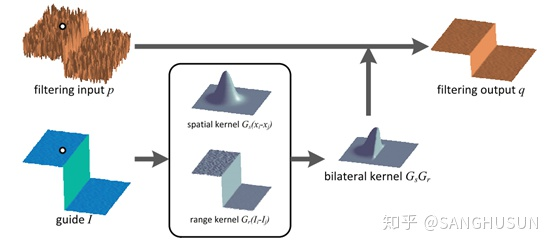这是来源于Kaiming He（何恺明教授）其文章中的原理图，其中的p为输入图像，其中的q为输出图像，I为输入的引导图像，其中引导图像经过一个双边滤波核与输入图像进行结合，最终获得输出的图像。

导向滤波其基本的算法原理可以见下图：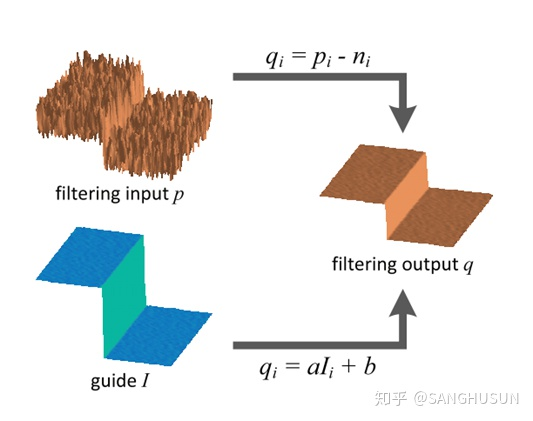其中输出图像q有：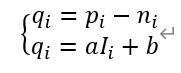其中的输出图像q又有如下表达：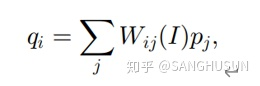我们可以看到的是Wij为权值，这个权值限定了图像q的输出，而这个权值的来源是引导图像I，所以W是一个与I有关的数值，且其为一个滤波核，其中i与j为图像的像素坐标引导，由此表达式我们可以直观的看出，输出图像和输入图像p，存在的是一种线性关系。

在导向滤波中，核心的假设在于，在一个以k为核心的处理窗口中，输出图像的q是一种线性的模式，也即：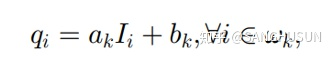其中的ak和bk为窗口中的常系数，同时我们需要设定一个正方形的窗格，此窗格的半径为r。同时ak和bk的来源，我们都通过上文得知，其由输入的图像来进行限制，我们认为，输出的图像是输入图像减去噪声而形成的，故有：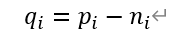其中的ni为图像中的噪声，而我们需要将此图像中的噪声降低到最低，所以需要找到一种比较好的方法来达到这一点：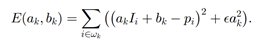我们可以看到上式为一个关于ak和bk的像素损失函数，同时我们又有一个调整参数，我们将调整参数设定为e，这个调整参数限定了ak。在何凯明教授之后的文中提到，此函数是一种脊回归模型，我们可以获取ak和bk其显式解为：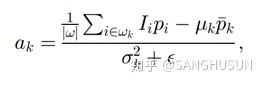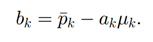其中uk为引导图I中的窗口中的像素均值，而为窗口中的像素方差，而下式的e

则为调整参数，而w为窗口的像素点的个数，而下式中的pk为：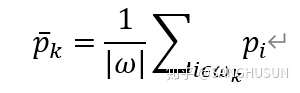那么当我们将此应用于具体的图像中时，对于一个N*N的处理窗格，一个像素点就会被包含N次，也即我们需要利用w，来进行取均值的处理：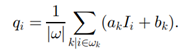同时我们注意到ak和bk，所以我们可以将上式改写为：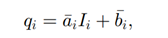这样我们就建立了一个，从p，再依据I，最后到q的一种映射的过程，这也就是导向滤波整体算法原理，而当我们的导向图I和输入图片为同一张图片时，此种滤波算法就具有边缘保持的能力。

我们总结一下此种算法即：其输入端有4个输入：

输入图像p，引导图像I，处理窗口半径r，调整参数e。

而其输出为一张图像：

输出图像q。

其具体的算法实现过程：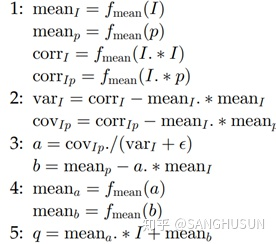我们利用matlab进行对原始的噪点较多的图形进行处理，首先我们是可以得知，此处我们输入图像和引导图像为同一张图像，此处我们设置三种窗口的规格

r0=2;r1=3;r2=4；

而对于调整参数，我们同样设置三种：

Eps0=0.01，eps1=0.04，eps2=0.09；

故对于此滤波器的调用方式为：

subplot(3,3,1);

gfout1_1=guidedfilter(gfI,gfp,r0,eps0);

imshow(gfout1_1);

title('r=2,eps=0.01');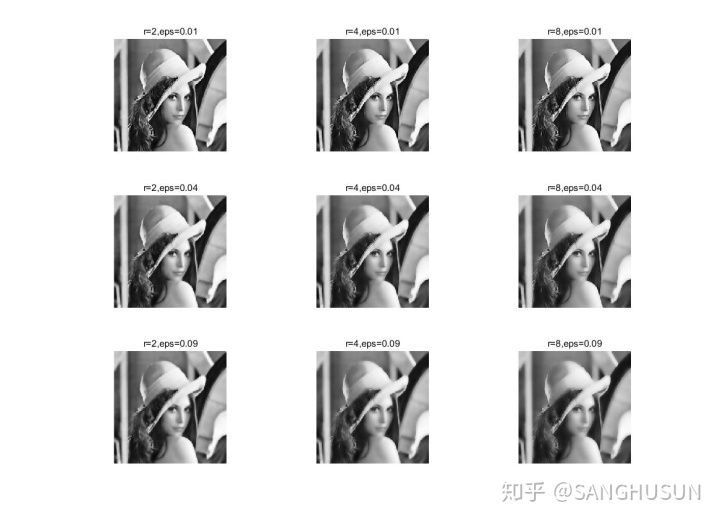Guidedfilter.h:
function q = guidedfilter(I, p, r, eps)

%   - guidance image: I (should be a gray-scale/single channel image)
%   - filtering input image: p (should be a gray-scale/single channel image)
%   - local window radius: r
%   - regularization parameter: eps

[hei, wid] = size(I);
N = boxfilter(ones(hei, wid), r);

mean_I = boxfilter(I, r) ./ N;
mean_p = boxfilter(p, r) ./ N;
mean_Ip = boxfilter(I.*p, r) ./ N;
% this is the covariance of (I, p) in each local patch.
cov_Ip = mean_Ip - mean_I .* mean_p;

mean_II = boxfilter(I.*I, r) ./ N;
var_I = mean_II - mean_I .* mean_I;

a = cov_Ip ./ (var_I + eps);
b = mean_p - a .* mean_I;

mean_a = boxfilter(a, r) ./ N;
mean_b = boxfilter(b, r) ./ N;

q = mean_a .* I + mean_b;
end
boxfilter.h:
function imDst = boxfilter(imSrc, r)

%   BOXFILTER   O(1) time box filtering using cumulative sum
%
%   - Definition imDst(x, y)=sum(sum(imSrc(x-r:x+r,y-r:y+r)));
%   - Running time independent of r;
%   - Equivalent to the function: colfilt(imSrc, [2*r+1, 2*r+1], 'sliding', @sum);
%   - But much faster.

[hei, wid] = size(imSrc);
imDst = zeros(size(imSrc));

%cumulative sum over Y axis
imCum = cumsum(imSrc, 1);
%difference over Y axis
imDst(1:r+1, :) = imCum(1+r:2*r+1, :);
imDst(r+2:hei-r, :) = imCum(2*r+2:hei, :) - imCum(1:hei-2*r-1, :);
imDst(hei-r+1:hei, :) = repmat(imCum(hei, :), [r, 1]) - imCum(hei-2*r:hei-r-1, :);

%cumulative sum over X axis
imCum = cumsum(imDst, 2);
%difference over Y axis
imDst(:, 1:r+1) = imCum(:, 1+r:2*r+1);
imDst(:, r+2:wid-r) = imCum(:, 2*r+2:wid) - imCum(:, 1:wid-2*r-1);
imDst(:, wid-r+1:wid) = repmat(imCum(:, wid), [1, r]) - imCum(:, wid-2*r:wid-r-1);
end

算法总体评价：导向滤波作为三大边缘保持滤波器之一，其平滑效果和边缘保持能力值得肯定，但是其同时因为其算法原因会产生光晕（halo）此点何恺明教授也在其文章中详细说明，其与另外两种边缘保持滤波算法相比，其一大特点就在于其输入，其输入为一张输入图像和一张引导图像，所以这点是一个非常鲜明的特点，同时需注意的是，要是其存在边缘保持的滤波效果，需要使引导图像和输入图像为同一张图像，而且其处理窗口和损失参数的设置对其滤波效果的影响较大，此点可详见上文。

参考文献

____________________________________________________________________________________________

• Guided Image Filtering, by Kaiming He, Jian Sun, and Xiaoou Tang, in TPAMI 2013.

SANGHUSUN

2020.02.10

展开全文• guide filter导向滤波算法的matlab代码，针对guide filter有几种方式的处理效果，对比之后可根据自己的情况选择最优方案。
• 混合滤波真棒深层HDR 基于深度学习的HDR图像合成方法的集合 多视图HDR图像合成 动态场景的深度高动态范围成像（ SIGGRAPH Asia 2017 ） 用于深度高动态范围成像的多尺度密集网络（ WACV 2019 ） ） 具有大前景运动的...
• 引导图滤波器是一种自适应权重滤波器，能够在平滑图像的同时起到保持边界的作用，具体公式推导请查阅原文献《Guided Image Filtering》以及matlab源码：http://kaiminghe.com/eccv10/index.html。这里只说一下自适应...
• 双边滤波器的定义 双边滤波器（Bilateral filter）为使...双边滤波器的好处是可以做边缘保护（edge preserving），一般用高斯滤波、均值滤波等滤波器去降噪，会较明显地模糊边缘，对于高频细节的保护效果并不明显...
• 导向滤波磨皮的对应文献为：Guided Image Filtering，这个算法速度极其之快，比其他的保边缘磨皮算法都快，甚至快上好几倍。这个算法最初来自于何明凯的图像去雾算法中，现在已然被应用封装与matlab图像处理函数库中...
• Kaiming He的导向滤波matlab代码
• 基本去噪滤波器存在的问题：各向同性滤波，比如box filter/Gaussian filter。这类滤波器在能够平滑噪声的影响的同时，也会抹去一些细节，减弱edge在图片中的表现。有时我们需要保留edge的feature，因此有一些人对...
• 滤波类型，可输入’cross’ 调用十字中值滤波 或 ‘average’ 调用均值滤波。 scale：整型。 滤波核大小， 必须是单数，如3，代表3×3的卷积核。 举例： close all;clear all;clc load('dip_pic.mat'); cross = img...
• 何恺明等人研究出的基于暗通道的经典图像去雾算法，不仅可以还原图像的颜色和能见度，同时也能利用雾的浓度来估计物体的距离。 (The classic fog removal algorithm based on dark channel, which was developed by ...
• 使用结构导向滤波处理三维地震数据(也可以处理三通道彩色图片) 代码链接 结构导向滤波 输入/输出 matlab .mat格式 代码环境 armadillo-9.600.6 / matlab C++混合编程 / 未使用openCV 数据维度 Inline x Xline x ...C++
• ## 导向滤波详解

万次阅读 2018-05-23 16:34:47
现在从一个最简单的情形来开始...滤波之后的图像为qq，如下图所示，图像qq中第ii个像素是由图像pp中以第ii个像素为中心的一个窗口ww中的像素确定的。具体而言，在简单平滑中，图像qq中第ii个像素是由图像pp中以第...
• ## 基于导向滤波的图像融合(GFF)

万次阅读 多人点赞 2019-07-15 18:09:00
论文学习——基于导向滤波的图像融合(GGF) 原文 Image Fusion with Guided Filtering - 2013 简介   本文提出了一种基于导向滤波的图像融合方法。融合的对象可以是多光谱图像，不同焦点的图像，不同曝光的...图像融合
• 对网上搜集的暗通道代码学习后，自行修改，有完全符合理论的代码也有速度快的代码，文件夹里有说明。都能运行放心下载。...

# 导向滤波matlab代码matlab 订阅